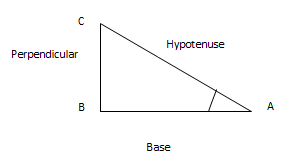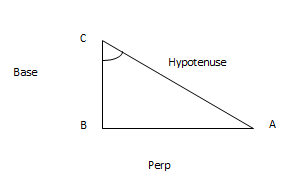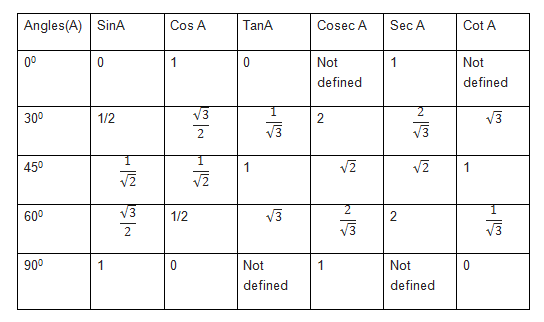# trigonometric formulas for Class 10 Maths

Trigonometry is quite a important chapter in Class 10 maths and lot of good questions comes in board examination also. Here is the trigonometric formulas for Class 10 in one page

In a right angle triangle ABC where B=90°We can define following term for angle A

Base :  Side adjacent to angle

Perpendicular: Side Opposite of angle

Hypotenuse: Side opposite to right angle

We can define the trigonometric ratios for angle A as

$sin A= \frac {Perpendicular}{Hypotenuse} =\frac {BC}{AC}$

$cosec A= \frac {Hypotenuse}{Perpendicular} =\frac {AC}{BC}$

$cos A= \frac {Base}{Hypotenuse} =\frac {AB}{AC}$

$sec A= \frac {Hypotenuse}{Base}=\frac {AC}{AB}$

$tan A= \frac {Perpendicular}{Base} =\frac {BC}{AB}$

$cot A= \frac {Base}{Perpendicular}=\frac {AB}{BC}$

Notice that each ratio in the right-hand column is the inverse, or the reciprocal, of the ratio in the left-hand column.

Reciprocal of functions

$cosec A = \frac {1}{sin A}$

$sec A = \frac {1}{cos A}$

$cot A =\frac {cos A }{sin A} = \frac {1}{tan A}$

Trigonometric ratio from complementary angle in Right angle triangle$sin C= \frac {Perpendicular}{Hypotenuse} =\frac {AB}{AC}$

$cosec C= \frac {Hypotenuse}{Perpendicular} =\frac {AC}{AB}$

$cos C= \frac {Base}{Hypotenuse} =\frac {BC}{AC}$

$sec C= \frac {Hypotenuse}{Base}=\frac {AC}{BC}$

$tan A= \frac {Perpendicular}{Base} =\frac {AB}{BC}$

$cot A= \frac {Base}{Perpendicular}=\frac {BC}{AB}$

This can also be written as C= 90 -A

$Sin (90-A) =cos(A)$

$Cos(90-A) = sin A$

$Tan(90-A) =cot A$

$Sec(90-A)= cosec A$

$Cosec (90-A) =sec A$

$Cot(90- A) =tan A$

Trigonometric identities

$Sin^2 A + cos^2 A =1$
$1 + tan^2 A =sec^2 A$
$1 + cot^2 A =cosec^2 A$

Trigonometric Ratios of standard anglesYou can below post on how to remember this easily

How to easily remember trigonometric ratios table

How to Find trigonometric ratios in case one is given

$sin A = \sqrt {1 – cos^2A}$

$cos A = \sqrt {1 – sin^2A}$

$tan A = \frac {sin A}{\sqrt {1 – sin^2A}} = \frac {1}{cot A} = \sqrt { sec^2 A -1} = \frac {1}{\sqrt { cosec^2 A -1} }$

$cot A = \frac {1}{tan A}$

$sec A = \sqrt { 1 + tan^2 A} = \frac {1}{cos A}$

$cosec A = \sqrt {1 + cot^2 A} = \frac {1}{sin A}$

Range of Values of trigonometric ratios

Values of Sin A and cos A is always less than or equal to 1

Values of sec A and cosec A is always greater than or equal to 1

Hope you like this post on trigonometric formulas for Class 10 Maths

Related Articles

Trigonometry Notes for Class10

Class 10 trigonometry Important Questions

Class 10 trigonometry worksheet